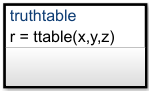## 使用真值表对组合逻辑建模

• 故障检测与管理

• 模式切换

• 如果想要在图的任意位置调用函数，需将真值表置于与图同一层次的位置。

• 如果想从一个状态（或子图）及其子状态内调用函数，只需将真值表置于该状态或子图中。该函数将覆盖该状态或子图的父级中同名的任何其他函数。

• 如果要从模型中的任何图调用该函数，请将真值表放在图级别，并启用图级别函数的导出。有关详细信息，请参阅Export Stateflow Functions for Reuse

### 真值表的布局`x == 1`

τ

F

F

-

`y == 1`

F

τ

F

-

`z == 1`

F

F

τ

-

`r = 1``r = 2``r = 3``r = 4`

```if ((x == 1) & !(y == 1) & !(z == 1)) r = 1; ```

```elseif (!(x == 1) & (y == 1) & !(z == 1)) r = 2; ```

```elseif (!(x == 1) & !(y == 1) & (z == 1)) r = 3; ```

```else r = 4; endif ```

### 定义真值表函数

1. 声明真值表函数参数和返回值。

2. 编写真值表函数。请参阅编写真值表

3. 使用 Property Inspector 和 Symbols 窗格为每个参数和返回值指定数据属性。

4. 使用 Symbols 窗格创建函数所需的任何附加数据项。您的函数可以访问它自己的数据或属于父状态或图的数据。

#### 声明函数参数和返回值

```[return_val1,return_val2,...] = function_name(arg1,arg2,...) ```

```[y1,y2,y3] = f(y1,u,y2) ```

### 指定真值表函数的属性

#### Function Inline Option

• `Auto`” - 确定是否根据内部计算内联函数。

• `Inline`” - 如果不将函数导出到其他图并且它不是递归的一部分，则内联函数。（如果函数直接调用自身或通过另一个函数调用间接调用自身，则存在递归。）

• `Function`” - 不内联函数。

## 支持